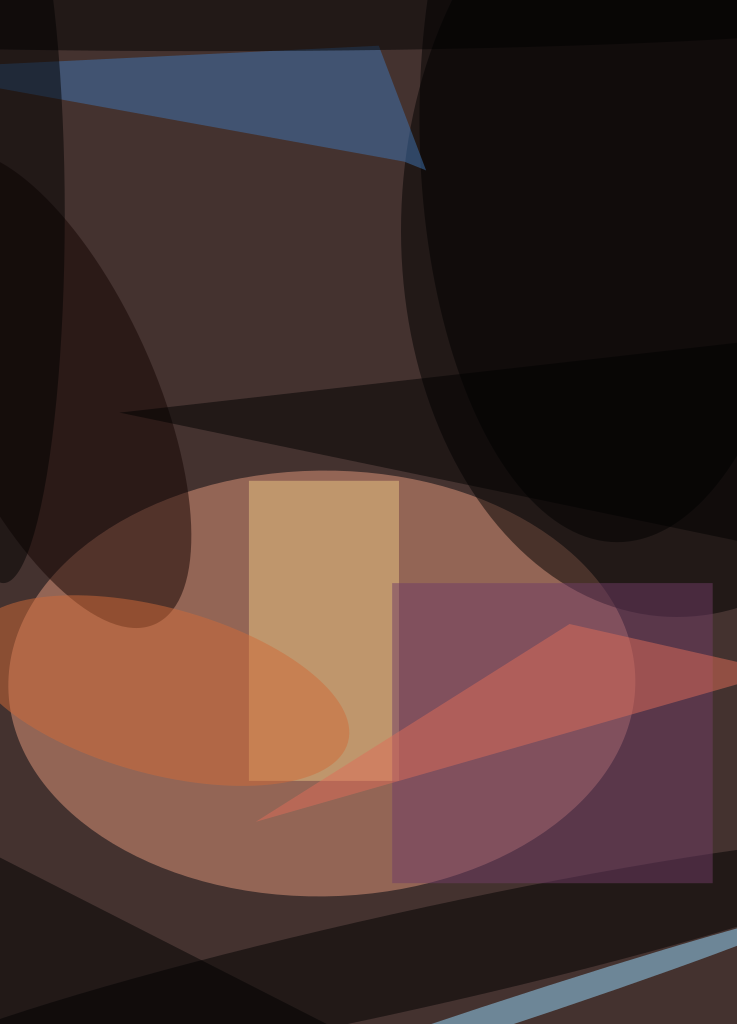A method of observing the interdiffusion of a pair of miscible liquids is based on the use of a common-path interferometer (CPI) to measure the spatially varying gradient of the index refraction in the interfacial region in which the interdiffusion takes place. Assuming that the indices of refraction of the two liquids are different and that the gradient of the index of refraction of the liquid is proportional to the gradient in the relative concentrations of either liquid, the diffusivity of the pair of liquids can be calculated from the temporal variation of the spatial variation of the index of refraction. This method yields robust measurements and does not require precise knowledge of the indices of refraction of the pure liquids. Moreover, the CPI instrumentation is compact and is optomechanically robust by virtue of its common-path design.

The two liquids are placed in a transparent rectangular parallelepiped test cell. Initially, the interface between the liquids is a horizontal plane, above which lies pure liquid 2 (the less-dense liquid) and below which lies pure liquid 1 (the denser liquid). The subsequent interdiffusion of the liquids gives rise to a gradient of concentration and a corresponding gradient of the index of refraction in a mixing layer. For the purpose of observing the interdiffusion, the test cell is placed in the test section of the CPI, in which a collimated, polarized beam of light from a low-power laser is projected horizontally through a region that contains the mixing layer.

The CPI used in this method is a shearing interferometer. Like other shearing interferometers, this CPI can also be characterized as a schlieren interferometer because its optical setup is partly similar to that of schlieren system. However, the basic principle of operation of this CPI applies to the case in which refraction is relatively weak so that unlike in a schlieren system, rays of light propagating through the test cell can be assumed not to be bent, but, rather, delayed (and correspondingly changed in phase) by amounts proportional to the indices of refraction along their paths. After passing through the test cell, the beam is focused on a Wollaston prism, which splits the beam into two beams that are slightly displaced from each other. When the beams are recombined, they produce interference fringes that indicate gradients of refraction in the test cell. A charge-coupled-device (CCD) camera captures the interferograms, and a video recorder stores them for later analysis.

The interferometer optics are arranged for operation in a mode, known in the art as the finite-fringe mode, in which equidistant, parallel interference fringes appear when the index of refraction in the test cell is uniform (as is the case when only one fluid is present). When a second liquid is introduced and diffusion occurs, the deviation or shift of a fringe from its undisturbed location is a measure of the gradient of the index of refraction in the test cell. For the purpose of this method, it is assumed that the index of refraction is horizontally uniform and varies only as a function of height above or below the initial interfacial plane.

An important element of the present method is rotation of the Wollaston prism around its optical axis by a small amount chosen so that the interference fringes form at a slight angle with respect to the initial interface between the liquids. The advantage of this angle is not intuitively obvious, and can be understood only in terms of the applicable equations. In summary, what the equations show is that proper choice of the angle results in magnification of the visual effect of the gradient of concentration in the mixing zone. Without the proper choice of the angle, the interference-fringe image cannot be interpreted simply or used to obtain the diffusivity of the fluids.

This work was done by Nasser Rashidnia of Glenn Research Center.

Inquiries concerning rights for the commercial use of this invention should be addressed to

###### NASA Glenn Research CenterCommercial Technology OfficeAttn: Steve FedorMail Stop 4-821000 Brookpark RoadClevelandOhio 44135

Refer to LEW-17375.##### Photonics Tech Briefs Magazine

This article first appeared in the November, 2003 issue of Photonics Tech Briefs Magazine.

Read more articles from the archives here.